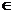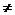2.11 Remainder Theorem:

We notice that the method mentioned in section 2.10 to find the remainder by repeated process of divisions, is a long process and is time consuming..

Is there a simple method by which remainder can be found?

By analyzing the problems solved in Section 2.10 we can observe a few things.

In case of the Problem 2.10.3.1, let us call dividend as f(a) {pronounced as function of a}then

f(a) = a3-6a +7

Let us find the values of f(a) for various values of a (say 1, 2,0,-1,-2)

Then we have

f(1) =  2, f(0) =7, f(-1) = 12, f(-2) = 11.

What do we observe? We notice f(-1)=12 is the remainder.

In case of the Problem 2.10.3.2, let us call dividend as f(x) (function of x) then

f(x) = x4-2x3+x-7

Let us find the value of f(x) for various vales of x (say 1, 2, 0,-1,-2)

Then we have

f(1) =  -6, f(2)  =-5,  f(0)  =-7, f(-1) =-7,  f(-2)=23  is the remainder

Let us do some tabulation of remainders for various values of dividends and divisors

 Dividend- f(x) Divisor Remainder Remainder= to value of function x3-6x +7 x+1 12 f(-1) x4-2x3+x-7 x+2 23 f(-2) x+1 x+1 0 f(-1) x-1 x-1 0 f(1) x+a x+a 0 f(-a) x-a x-a 0 f(a) x2+4x+4 x+2 0 f(-2)

We conclude that if a polynomial f(x) is divided by a monomial of type (x+a) then the remainder is equal to f(-a)

This is called Remainder Theorem:

If a Polynomial f(x), over the set of real numbers R  is divided by (x+a)  then the remainder is f(-a).

Note: “over the set of real numbers” means that x can take the value of any real number (xreal number)

2.11 Problem 1: Find remainder of   x3+2x2-x+6 when divided by x-3

Solution:

Here f(x) = x3+2x2-x+6 and divisor is x-3

As per the remainder theorem, if divisor is of the form x+a then the remainder is f(-a).

Since divisor is of the form x+a , f(-(-3) = f(3) is the remainder

Substituting 3 in f(x), we get

f(3) = 27+ 18-3+6 = 48 is the remainder

2.11 Problem 2: Find value of a if x+2 is a factor of 4x4+2x3-3x2+8x+5a

Solution:

Since x+2 is a factor of f(x), remainder has to be 0.

Thus by Remainder theorem f(-2) =0

f(-2) = 4*16+2*(-8)-3*4 -16+5a = 64-16-12-16+5a = 20 +5a

Since f(-2) =0 we have 20+5a = 0 i.e. 5a = -20 i.e. a= -4

Verification:

Since a= -4, substitute -4 for a in f(x)

We get f(x) = 4x4+2x3-3x2+8x-20

f(-2)  = 4*16+2(-8)-3*4 -16 -20 = 64-16-12-16-20 = 0

This proves that x+2 is a factor of 4x4+2x3-3x2+8x-20

2.11 Summary of learning

 No Points studied 1 If a polynomial f(x) is divided by (x+a) then the remainder = f(-a) for any real value of x 2 If a polynomial f(x) is divided by the divisor of the form x+a and the remainder is zero, then  x+a is a factor of f(x)

Proof of Remainder theorem:

Remainder Theorem:

If a Polynomial f(x), is divided by (x+a) then the remainder is f(-a).

Let q(x) and r(x) be the quotient and the remainder when we divide f(x) by x+a.

Proof:

We have Dividend = Quotient*Divisor + Remainderf(x) = q(x)*(x+a) + r(x)

Note that the degree of the divisor (=(x+a)) is 1.

Also note that the degree of remainder (= r(x)) has to be less than the degree of divisor.

Hence, the degree of remainder = 0 which means that r(x) does not contain the term x and so it has to be a constant (say ‘r’).f(x) = q(x)*(x+a)+r

Since the above equation is true for any value of x, it has to be true for x = -a also.f(-a) = q(-a)*(-a+a)+r = q(-a)*0+r = r

This proves the theorem.

Conversely

Factor Theorem:

(x+a) is a factor of the polynomial f(x) if the remainder of f(-a) is equal to 0 and conversely if f(-a) = 0 then (x+a) is a factor of f(x).

Proof:

Suppose f(-a) = 0

By remainder theorem, f(-a) is the remainder we get on dividing f(x) by x+a. So from the fact that f(-a)=0, it follows that the remainder on dividing f(x) by x+a = 0.This means that x+a divides f(x) exactly: that is x+a is a factor of f(x).

2.11 Problem 3: Check whether 7+3x is a factor of 3x3+7x

Solution:

Let f(x) = 3x3+7x

If 7+3x has to be a factor of f(x), then 3*(7/3+x) has to be necessarily a factor of f(x) (If y is a factor of f(x) then ny/n has to be a factor of f(x) as y*f(x) = (ny/n)*f(x) when n is any non zero number)f(-7/3) = 3(-7/3)3 +7(-7/3) =  -343/9 -49/30

Thus 7+3x is not a factor of the given polynomial.

Note: 3x3+7x = x(3x2+7) which clearly indicates that 7+3x is not a factor.

2.11 Problem 4: Factorise 6x2-11x+3

Solution:

Let f(x) = 6x2-11x+3

By factorisation method we find that the roots of the equation f(x) = 0 are 1/3 and 3/2

Hence (x-1/3) and (x-3/2) are factors of f(x)

(x-1/3)*(x-3/2)

= x2 - (1/3)x (3/2)x + 3/6

= x2 - (11/6)x +3/6

= (6x2-11x+3)/6.

Multiply both sides by 6 we getf(x) = 6x2-11x+3 = 6(x-1/3)*(x-3/2)

2.11 Problem 5: If (x+1) is a factor of x3 +2x2 - 5x – 6, find its other factors.

Hint:

Let f(x) = x3 + 2x2- 5x - 6

We also note that f(-1) = -1+2+5-6 = 0 thus clearly (x+1) is a factor of f(x).

Using the division method learnt in section 2.10, we find that

f(x) = (x+1)(x2+x-6)

By factorizing,

(x2+x-6)

= (x2+3x-2x-6)

= x(x+3)-2(x+3)

= (x+3)(x-2)

Hence

f(x) = (x+1)(x-2)(x+3)

2.11 Problem 6: If a quadratic polynomial, when divided by (x-1), (x+1) and (x-2) leaves the remainders 2, 4 and 4 respectively, find the quadratic equation.

Solution:

Let the quadratic polynomial be f(x) = ax2+bx+c

From the given data f(1)=2, f(-1)=4 and f(2)=4

But f(1) = a+b+c, f(-1) = a–b+c and f(2) = 4a+2b+cWe have

a+b+c = 2

a-b+c = 4

4a+2b+c = 4

When we solve these three simultaneous equations as explained in 2.14.3 we get

a=1, b=-1 and c=2

Hence the quadratic equation is x2-x+2.

2.11 Problem 7: Given that px2+qx+6 leaves a remainder of 1 when divided by 2x+1 and 2qx2+6x+p leaves a remainder of 2 when divided by 3x-1, find p and q.

Solution:

Let  f(x) = px2+qx+6, g(x) = 2qx2+6x+p

When f(x) is divided by 2x+1, the remainder is 1. This implies that f(-1/2) = 1p/4 –q/2+6 = 1

i.e. p-2q = -20 (On simplification)    ---à(1)

When g(x) is divided by 3x-1, the remainder is 2. This implies that g(1/3) = 22q/9 + 6/3 +p = 2

i.e. 9p+2q = 0 (On simplification)      ---à(2)

On solving (1) and (2) we get

p = -2 and q = 9